# The GAMPL Procedure

### Tests for Smoothing Components

The GAMPL procedure performs a smoothing component test on the null hypotheses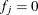for the jth component. In contrast to the analysis of deviance that is used in PROC GAM (which tests existence of nonlinearity for each smoothing component), the smoothing component test used in PROC GAMPL tests for the existence of a contribution for each smoothing component.

The hypothesis test is based on the Wald statistic. Define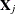as the matrix of all zeros except for columns that correspond to basis expansions of the jth spline term. Then the column vector of predictions is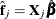, and the covariance matrix for the predictions is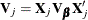. The Wald statistic for testing is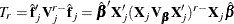where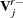is the rank-r pseudo-inverse of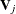. If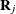is the Cholesky root for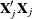such that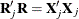, then the test statistic can be written as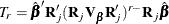Wood (2012) proposes using the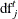degrees of freedom for test (which is defined in the section Degrees of Freedom) as the rank r. Because spline terms in fitted models often have noninteger degrees of freedom, the GAMPL procedure uses a rounded value ofas the rank: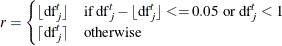Let K be a symmetric and nonnegative definite matrix, and let its eigenvalues be sorted as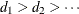; then the rank-r pseudo-inverse of K is formed by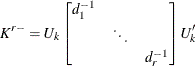where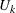are formed by columns of eigenvectors that correspond to the r eigenvalues.

Under the null hypothesis, the Wald statistic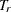approximately follows the chi-square distribution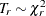. For an observed test statistic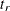, the p-value for rejecting the null hypothesis is computed as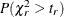if the dispersion parameter is constant, or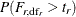with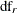error degrees of freedom if the dispersion parameter is estimated.

Be cautious when you interpret the results of the smoothing component test because p-values are computed by approximation and the test does not take the smoothing parameter selection process into account.